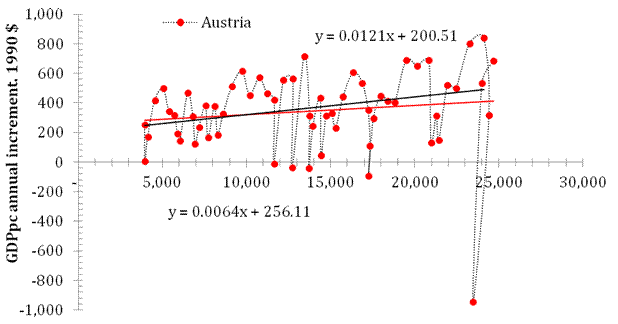## Growth Rate Of The GDP Per Capita Revisited. The Results From 2007, 2009, And 2012 Revisited

Growth rate of the GDP per capita revisited. 3. The results from 2007, 2009, and 2012 revisited. The model

GDP per capita data revisited

We start with a revision of the period after 1950. Originally, we estimated the evolution of annual increment before 2003. Then data for the period between 2004 and 2007 were added the prediction of the model was estimated. In 2012, 4 new readings were added for all involved countries. In 2006, we made a model-based assumption (see Appendix in this post for the model description) that all large deviations from the linear trend in the annual GDP per capita should fade away in the near future. In this post, we claim that this assumption was an extremely successful one and it statistically validates the model of linear GDP per capita growth.

The upper panel of Figure 1 is borrowed from the 2012 paper and presents the comparison of GDP per capita in Austria for the period between 1950 and 2011. The positive slope reported in 2008 (black line, slope=0.012 \$/\$) decreased between 2007 and 2011 (red line, slope=0.0064 \$/\$), as predicted. Since 2007, the period of low economic performance with an extended recession resulted in a further decline in the speed of economic growth and the lower panel of Figure 1 shows that the slope (the MPD data are used for this analysis) fell to 0.0014 \$/\$. In 2018, the linear regression (black) line is not much different from the mean value (red dotted) line. Figure 2 presents a similar analysis made for the USA where the slope for the period between 1951 and 2018 is close to that observed for the period between 1950 and 2011 because the major fall in economic growth was between 2008 and 2010. The mean value between 1951 and 2018 is \$569, i.e. slightly higher than that in Austria.Figure 1. The upper panel: annual increment of real GDP per capita (in 1990 US dollars) as a function of real GDP per capita in Austria for the period between 1951 and 2011. The regression (red) line slope is \$0.0064 per dollar. For the period between 1951 and 2007, the regression (black) line has a larger slope of \$0.012 per dollar. The lower panel: Same as in the upper panel for the period between 1951 and 2018. The slope fell to \$0.014 per \$ (2011 prices).  The mean GDPpc annual increment value is \$547.4.Figure 2. Same as in Figure 1 for the USA. The slope for the period between 1951 and 2018 is close to that observed for the period between 1950 and 2011 because the major fall in economic growth was between 2008 and 2010. The mean value between 1951 and 2018 is \$569, i.e. slightly higher than that in Austria.

In the future post, we will revisit other countries and present the evolution of annual GDPpc increment between 1960 and 2018. The change is the studied period is justified in the previous post. Our principal aim is to prove that the model we have developed accurately predicts the economic growth in developed countries. Moreover, it gives an unbiased and theoretically justified view on the current rate of relative growth in GDPpc in various countries depending on the GDPpc level. One should not compare the relative rate of economic growth in China (GDPpc is \$13102 in 2018) and in the USA (GDPpc=\$55335 in 2018). One should compare the annual increments in the GDPpc and corresponding rates predicted by the model for inertial economic growth. In that sense, the USA growth rate is much higher than that observed in China. However, the population in China is 4 times larger than in the USA and this gives an impression of faster economic growth in the former.

Disclosure: None.

How did you like this article? Let us know so we can better customize your reading experience. Users' ratings are only visible to themselves.